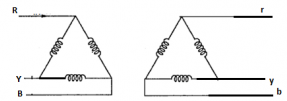Courses

# Test: Three-Phase Transformers

## 10 Questions MCQ Test Electrical Machines | Test: Three-Phase Transformers

Description
This mock test of Test: Three-Phase Transformers for Electrical Engineering (EE) helps you for every Electrical Engineering (EE) entrance exam. This contains 10 Multiple Choice Questions for Electrical Engineering (EE) Test: Three-Phase Transformers (mcq) to study with solutions a complete question bank. The solved questions answers in this Test: Three-Phase Transformers quiz give you a good mix of easy questions and tough questions. Electrical Engineering (EE) students definitely take this Test: Three-Phase Transformers exercise for a better result in the exam. You can find other Test: Three-Phase Transformers extra questions, long questions & short questions for Electrical Engineering (EE) on EduRev as well by searching above.
QUESTION: 1

### A V-V connected transformer can be connected in parallel to delta-delta connected transformer but not to

Solution:

The V-V connected transformer and D-D connected transformers have same phase displacement, so they only can be connected in parallel to each other.

QUESTION: 2

### Three units of single phase transformers and one single three-phase transformer rating

Solution:

Three single phase transformers and one single unit of three phase transformer will be same only. this is done to reduce the cost and spacing.

QUESTION: 3

### The transformer which is more feasible to use in the distribution ends should be

Solution:

Star-delta will have lower voltage at delta end.

QUESTION: 4

The delta-delta connections are used in applications of

Solution:

Delta connected winding handle line voltages so it needs more turns in the winding but thin wires.

QUESTION: 5

Open delta transformers can be obtained from

Solution:

If one of the transformers is removed from the bank of only delta-delta, then it behaves with 58% power delivery.

QUESTION: 6

If para magnetic core is used in the place of the ferromagnetic core of the transformer, then magnetostriction will

Solution:

If the core is not ferromagnetic then the transformer will not operate at all so no humming sound.

QUESTION: 7

When we magnetize the ferromagnetic core of the transformer, core length_______ and it ______ when demagnetized.

Solution:

Due to aligned magnetic dipoles in the material, the size will reduce by a small margin and vice versa while demagnetization.

QUESTION: 8

A 400 V, 10 KVA transformer at 50 Hz, is operated at the frequency of 40 Hz, then the humming

Solution:

If the frequency is reduced, the core flux density increases, so the noise also will increase.

QUESTION: 9

A recording of the output of the emf induced for star and delta are recorded.Then shape of the emf induced in Y-connected 3-phase transformer is non sinusoidal in nature due to

Solution:

In a star connected transformer, closed path to generate 3rd harmonic is not there. So the emf shape will be peaky in nature.

QUESTION: 10

Below is the circuit diagram for delta-delta transformer have a transformation ratio ‘k’. The current flowing in the windings of phase r-y in the below diagrams isSolution:

The phase current will be reduced by 1/1.73.